# Develop Visual SLAM Algorithm Using Unreal Engine Simulation

This example shows how to develop a visual Simultaneous Localization and Mapping (SLAM) algorithm using image data obtained from the Unreal Engine® simulation environment.

Visual SLAM is the process of calculating the position and orientation of a camera with respect to its surroundings while simultaneously mapping the environment. Developing a visual SLAM algorithm and evaluating its performance in varying conditions is a challenging task. One of the biggest challenges is generating the ground truth of the camera sensor, especially in outdoor environments. The use of simulation enables testing under a variety of scenarios and camera configurations while providing precise ground truth.

This example demonstrates the use of Unreal Engine simulation to develop a visual SLAM algorithm for either a monocular or a stereo camera in a parking scenario. For more information about the implementation of the visual SLAM pipelines, see the Monocular Visual Simultaneous Localization and Mapping example and the Stereo Visual Simultaneous Localization and Mapping example.

### Set Up Scenario in Simulation Environment

Use the Simulation 3D Scene Configuration block to set up the simulation environment. Select the built-in Large Parking Lot scene, which contains several parked vehicles. The visual SLAM algorithm matches features across consecutive images. To increase the number of potential feature matches, you can use the Parked Vehicles subsystem to add more parked vehicles to the scene. To specify the parking poses of the vehicles, use the `helperAddParkedVehicle` function. If you select a more natural scene, the presence of additional vehicles is not necessary. Natural scenes usually have enough texture and feature variety suitable for feature matching.

You can follow the Select Waypoints for Unreal Engine Simulation (Automated Driving Toolbox) example to interactively select a sequence of parking locations. You can use the same approach to select a sequence of waypoints and generate a reference trajectory for the ego vehicle. This example uses a recorded reference trajectory and parked vehicle locations.

```% Load reference path data = load("parkingLotReferenceData.mat"); % Set reference trajectory of the ego vehicle refPosesX = data.refPosesX; refPosesY = data.refPosesY; refPosesT = data.refPosesT; % Set poses of the parked vehicles parkedPoses = data.parkedPoses; % Display the reference path and the parked vehicle locations sceneName = "LargeParkingLot"; hScene = figure; helperShowSceneImage(sceneName); hold on plot(refPosesX(:,2), refPosesY(:,2), LineWidth=2, DisplayName='Reference Path'); scatter(parkedPoses(:,1), parkedPoses(:,2), [], 'filled', DisplayName='Parked Vehicles'); xlim([-60 40]) ylim([10 60]) hScene.Position = [100, 100, 1000, 500]; % Resize figure legend hold off```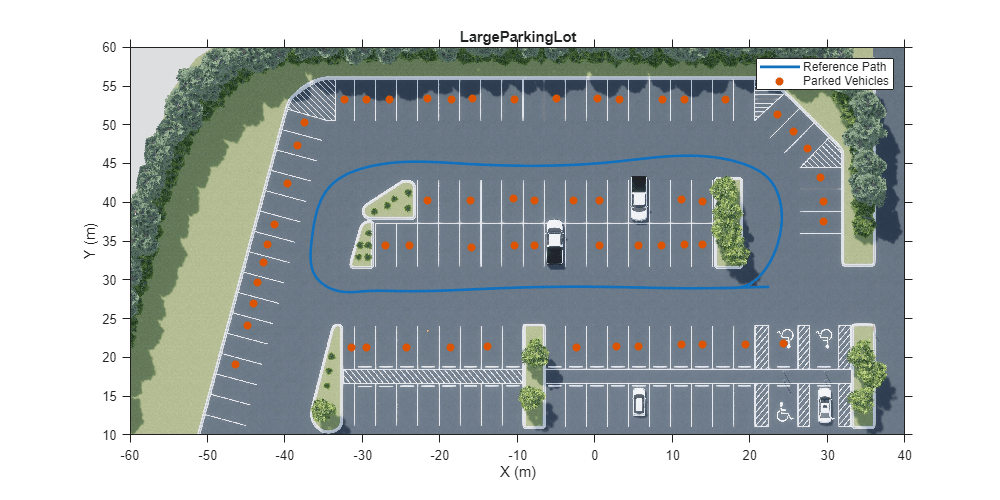Open the model and add parked vehicles

```modelName = 'GenerateImageDataOfParkingLot'; open_system(modelName);```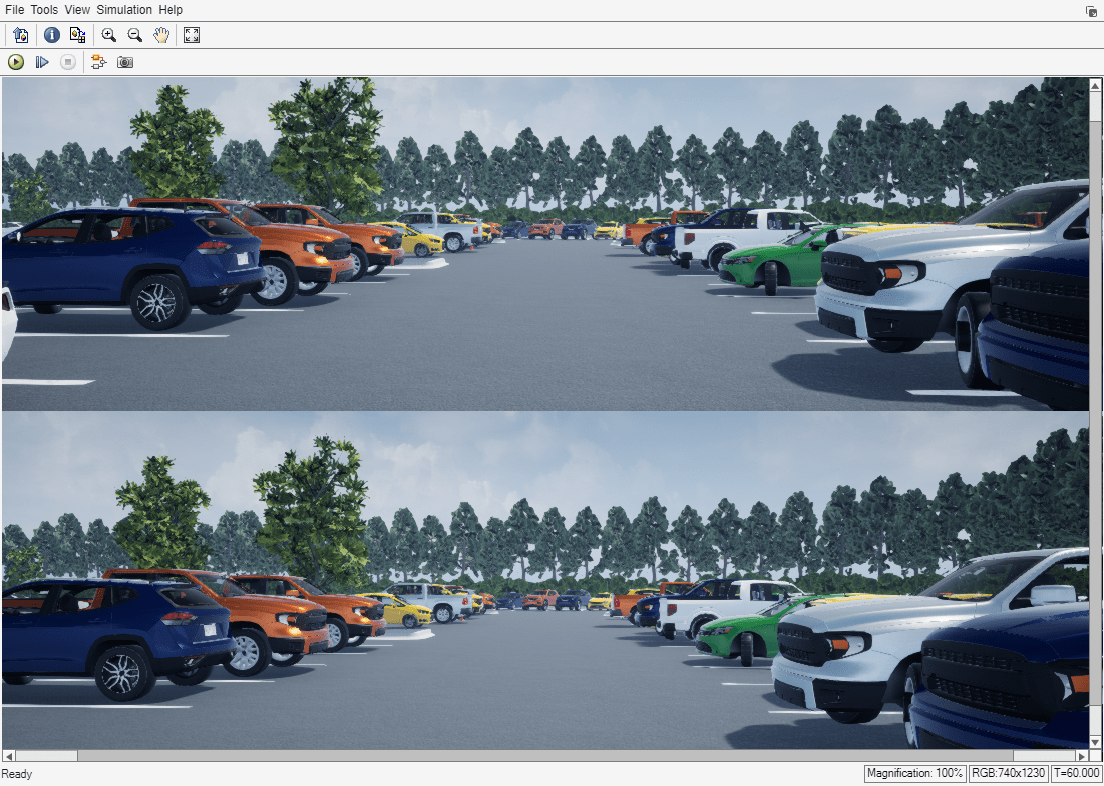`helperAddParkedVehicles(modelName, parkedPoses);`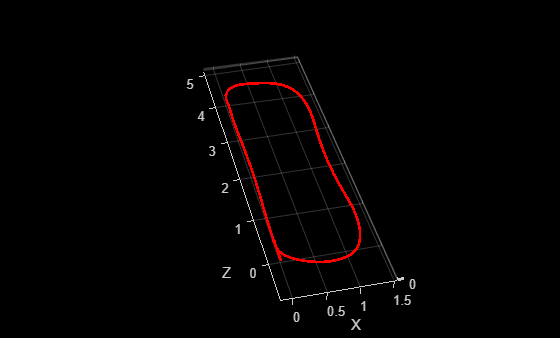### Set Up Ego Vehicle and Camera Sensor

Set up the ego vehicle moving along the specified reference path by using the Simulation 3D Vehicle with Ground Following block. The Camera Variant Subsystem contains two configurations of camera sensors: monocular and stereo. In both configurations, the camera is mounted on the vehicle roof center. You can use the Camera Calibrator or Stereo Camera Calibrator app to estimate intrinsics of the actual camera that you want to simulate. This example shows the monocular camera workflow first followed by the stereo camera workflow.

```% Select monocular camera useMonoCamera = 1; % Inspect the monocular camera open_system([modelName, '/Camera/Monocular']); % Camera intrinsics focalLength = [700, 700]; % specified in units of pixels principalPoint = [600, 180]; % in pixels [x, y] imageSize = [370, 1230]; % in pixels [mrows, ncols] intrinsics = cameraIntrinsics(focalLength, principalPoint, imageSize);```

### Visualize and Record Sensor Data

Run the simulation to visualize and record sensor data. Use the Video Viewer block to visualize the image output from the camera sensor. Use the To Workspace block to record the ground truth location and orientation of the camera sensor.

```close(hScene) if ~ispc error("Unreal Engine Simulation is supported only on Microsoft" + char(174) + " Windows" + char(174) + "."); end % Run simulation simOut = sim(modelName);```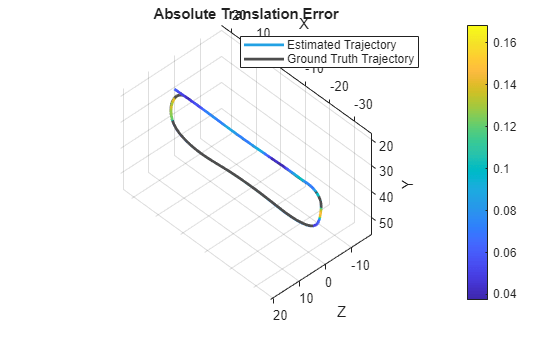```% Extract camera images as an imageDatastore imds = helperGetCameraImages(simOut); % Extract ground truth as an array of rigidtform3d objects gTruth = helperGetSensorGroundTruth(simOut);```

### Develop Monocular Visual SLAM Algorithm Using Recorded Data

Use the images to evaluate the monocular visual SLAM algorithm. The function `helperVisualSLAM` implements the monocular ORB-SLAM pipeline:

• Map Initialization: ORB-SLAM starts by initializing the map of 3-D points from two images. Use `estrelpose` to compute the relative pose based on 2-D ORB feature correspondences and `triangulate` to compute the 3-D map points. The two frames are stored in an `imageviewset` object as key frames. The 3-D map points and their correspondences to the key frames are stored in a `worldpointset` object.

• Tracking: Once a map is initialized, for each new image, the function `helperTrackLastKeyFrame` estimates the camera pose by matching features in the current frame to features in the last key frame. The function `helperTrackLocalMap` refines the estimated camera pose by tracking the local map.

• Local Mapping: The current frame is used to create new 3-D map points if it is identified as a key frame. At this stage, `bundleAdjustment` is used to minimize reprojection errors by adjusting the camera pose and 3-D points.

• Loop Closure: Loops are detected for each key frame by comparing it against all previous key frames using the bag-of-features approach. Once a loop closure is detected, the pose graph is optimized to refine the camera poses of all the key frames using the `optimizePoseGraph` (Navigation Toolbox) function.

For the implementation details of the algorithm, see the Monocular Visual Simultaneous Localization and Mapping example.

`[mapPlot, optimizedPoses, addedFramesIdx] = helperVisualSLAM(imds, intrinsics); `
```Map initialized with frame 1 and frame 4 ```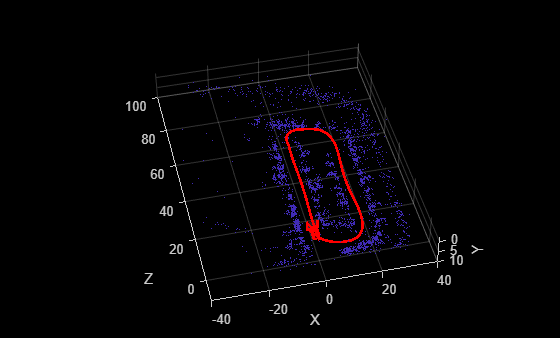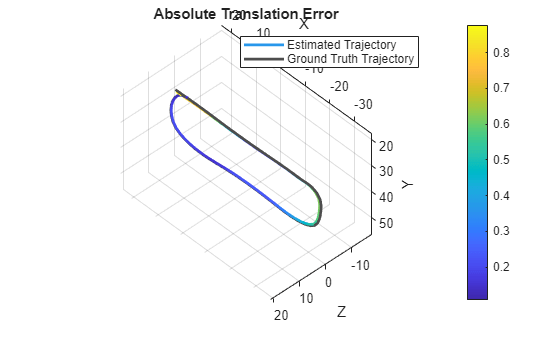```Loop edge added between keyframe: 4 and 97 ```### Evaluate Against Ground Truth

You can evaluate the optimized camera trajectory against the ground truth obtained from the simulation. Since the images are generated from a monocular camera, the trajectory of the camera can only be recovered up to an unknown scale factor. You can approximately compute the scale factor from the ground truth, thus simulating what you would normally obtain from an external sensor.

```% Plot the camera ground truth trajectory scaledTrajectory = plotActualTrajectory(mapPlot, gTruth(addedFramesIdx), optimizedPoses); % Show legend showLegend(mapPlot);```You can also calculate the root mean square error (RMSE) of trajectory estimates.

`helperEstimateTrajectoryError(gTruth(addedFramesIdx), scaledTrajectory);`
```Absolute RMSE for key frame trajectory (m): 1.9101 ```

### Stereo Visual SLAM Algorithm

In a monocular visual SLAM algorithm, depth cannot be accurately determined using a single camera. The scale of the map and of the estimated trajectory is unknown and drifts over time. Additionally, because map points often cannot be triangulated from the first frame, bootstrapping the system requires multiple views to produce an initial map. Using a stereo camera solves these problems and provides a more reliable visual SLAM solution. The function `helperVisualSLAMStereo` implements the stereo visual SLAM pipeline. The key difference from the monocular pipeline is that at the map initialization stage, the stereo pipeline creates 3-D map points from a pair of stereo images of the same frame, instead of creating them from two images of different frames. For the implementation details of the algorithm, see the Stereo Visual Simultaneous Localization and Mapping example.

```% Select stereo camera useMonoCamera = 0; % Inspect the stereo camera open_system([modelName, '/Camera/Stereo']); snapnow; % Set stereo camera baseline baseline = 0.5; % In meters % Construct the reprojection matrix for 3-D reconstruction reprojectionMatrix = [1, 0, 0, -principalPoint(1); 0, 1, 0, -principalPoint(2); 0, 0, 0, focalLength(1); 0, 0, 1/baseline, 0]; % Maximum disparity in stereo images maxDisparity = 48; % Run simulation simOut = sim(modelName);````snapnow;`

### Extract Stereo Images

```[imdsLeft, imdsRight] = helperGetCameraImagesStereo(simOut); % Extract ground truth as an array of rigidtform3d objects gTruth = helperGetSensorGroundTruth(simOut);```

Run the stereo visual SLAM algorithm

`[mapPlot, optimizedPoses, addedFramesIdx] = helperVisualSLAMStereo(imdsLeft, imdsRight, intrinsics, maxDisparity, reprojectionMatrix);````Loop edge added between keyframe: 2 and 92 ```
```% Plot the camera ground truth trajectory optimizedTrajectory = plotActualTrajectory(mapPlot, gTruth(addedFramesIdx)); % Show legend showLegend(mapPlot);``````% Calculate the root mean square error (RMSE) of trajectory estimates helperEstimateTrajectoryError(gTruth(addedFramesIdx), optimizedTrajectory);```
```Absolute RMSE for key frame trajectory (m): 0.27679 ```

Compared with the monocular visual SLAM algorithm, the stereo visual SLAM algorithm produces a more accurate estimation of the camera trajectory.

### Dense Reconstruction from Stereo Images

Given the refined camera poses, you can perform dense reconstruction from the stereo images corresponding to the key frames.

```pointCloudsAll = helperDenseReconstructFromStereo(imdsLeft, imdsRight, ... imageSize, addedFramesIdx, optimizedPoses, maxDisparity, reprojectionMatrix); % Visualize the scene figure(Position=[1100 600 1000 500]); ax = pcshow(pointCloudsAll,VerticalAxis="y", VerticalAxisDir="down"); xlabel('X') ylabel('Y') zlabel('Z') % Display bird's eye view of the parking lot view(ax, [0 -1 0]); camroll(ax, 90);```Close model and figures.

```close_system(modelName, 0); close all```

### Supporting Functions

helperGetCameraImages Get camera output

```function imds = helperGetCameraImages(simOut) % Save image data to a temporary folder dataFolder = fullfile(tempdir, 'parkingLotImages', filesep); folderExists = exist(dataFolder, 'dir'); if ~folderExists mkdir(dataFolder); end files = dir(dataFolder); if numel(files) < 3 numFrames = numel(simOut.images.Time); for i = 3:numFrames % Ignore the first two frames img = squeeze(simOut.images.Data(:,:,:,i)); imwrite(img, [dataFolder, sprintf('%04d', i-2), '.png']) end end % Create an imageDatastore object to store all the images imds = imageDatastore(dataFolder); end```

helperGetCameraImagesStereo Get camera output

```function [imdsLeft, imdsRight] = helperGetCameraImagesStereo(simOut) % Save image data to a temporary folder dataFolderLeft = fullfile(tempdir, 'parkingLotStereoImages', filesep, 'left', filesep); dataFolderRight = fullfile(tempdir, 'parkingLotStereoImages', filesep, 'right', filesep); folderExists = exist(dataFolderLeft, 'dir'); if ~folderExists mkdir(dataFolderLeft); mkdir(dataFolderRight); end files = dir(dataFolderLeft); if numel(files) < 3 numFrames = numel(simOut.imagesLeft.Time); for i = 3:numFrames % Ignore the first two frames imgLeft = squeeze(simOut.imagesLeft.Data(:,:,:,i)); imwrite(imgLeft, [dataFolderLeft, sprintf('%04d', i-2), '.png']) imgRight = squeeze(simOut.imagesRight.Data(:,:,:,i)); imwrite(imgRight, [dataFolderRight, sprintf('%04d', i-2), '.png']) end end % Use imageDatastore objects to store the stereo images imdsLeft = imageDatastore(dataFolderLeft); imdsRight = imageDatastore(dataFolderRight); end```

helperGetSensorGroundTruth Save the sensor ground truth

```function gTruth = helperGetSensorGroundTruth(simOut) numFrames = numel(simOut.location.Time); gTruth = repmat(rigidtform3d, numFrames-2, 1); for i = 1:numFrames-2 % Ignore the first two frames gTruth(i).Translation = squeeze(simOut.location.Data(:, :, i+2)); % Ignore the roll and the pitch rotations since the ground is flat yaw = double(simOut.orientation.Data(:, 3, i+2)); gTruth(i).R = [cos(yaw), -sin(yaw), 0; ... sin(yaw), cos(yaw), 0; ... 0, 0, 1]; end end```

helperEstimateTrajectoryError Calculate the tracking error

```function rmse = helperEstimateTrajectoryError(gTruth, scaledLocations) gLocations = vertcat(gTruth.Translation); rmse = sqrt(mean( sum((scaledLocations - gLocations).^2, 2) )); disp(['Absolute RMSE for key frame trajectory (m): ', num2str(rmse)]); end```

helperDenseReconstructFromStereo Perform dense reconstruction from stereo images with known camera poses

```function pointCloudsAll = helperDenseReconstructFromStereo(imdsLeft, imdsRight, ... imageSize, addedFramesIdx, optimizedPoses, maxDisparity, reprojectionMatrix) ptClouds = repmat(pointCloud(zeros(1, 3)), numel(addedFramesIdx), 1); for i = 1: numel(addedFramesIdx) I1 = readimage(imdsLeft, addedFramesIdx(i)); I2 = readimage(imdsRight, addedFramesIdx(i)); disparityMap = disparitySGM(im2gray(I1), im2gray(I2), DisparityRange=[0, maxDisparity],UniquenessThreshold=20); xyzPoints = reconstructScene(disparityMap, reprojectionMatrix); % Ignore the upper half of the images which mainly show the sky xyzPoints(1:100, :, :) = NaN; xyzPoints = reshape(xyzPoints, [imageSize(1)*imageSize(2), 3]); validIndex = xyzPoints(:, 3) > 0 & xyzPoints(:, 3) < 40/reprojectionMatrix(4, 3); xyzPoints = xyzPoints(validIndex, :); colors = reshape(I1, [imageSize(1)*imageSize(2), 3]); colors = colors(validIndex, :); currPose = optimizedPoses.AbsolutePose(i); xyzPoints = transformPointsForward(currPose, xyzPoints); ptCloud = pointCloud(xyzPoints, Color=colors); ptClouds(i) = pcdownsample(ptCloud, random=0.2); end % Concatenate the point clouds pointCloudsAll = pccat(ptClouds); end```